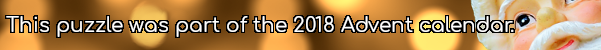mscroggs.co.uk
mscroggs.co.uksubscribe

# Advent calendar 2018

## Archive

Show me a random puzzle
▼ show ▼

## 18 DecemberThere are 6 terms in the expansion of $$(x+y+z)^2$$:
$$(x+y+z)^2=x^2+y^2+z^2+2xy+2yz+2xz$$
Today's number is number of terms in the expansion of $$(x+y+z)^{16}$$.
Tags: algebra
© Matthew Scroggs 2019